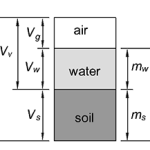## How to Calculate Degree of SaturationThe degree of saturation is the ratio of the volume of water in a soil to the volume of voids. Where: S = Degree of Saturation Vw = Volume of water (m3 or ft3) Vv = Volume of voids (m3 or ft3) Vg = Volume of air (m3 or ft3) The degree of saturation is […]